# 3 4 Find and Use Slopes of Lines

• Slides: 11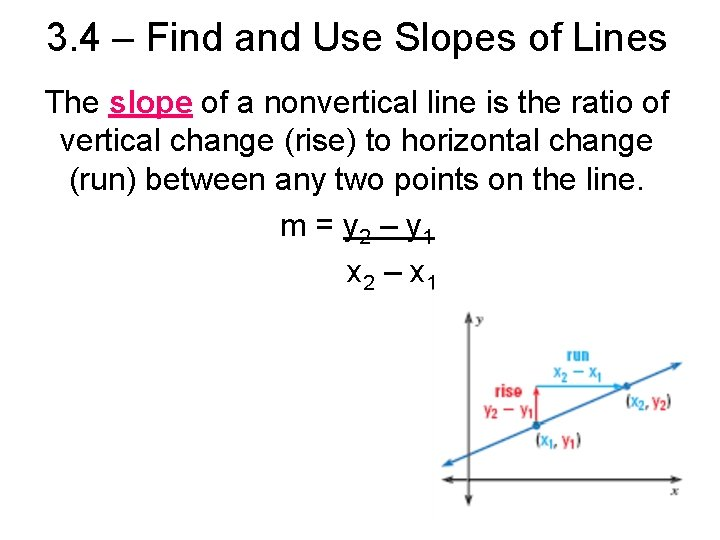3. 4 – Find and Use Slopes of Lines The slope of a nonvertical line is the ratio of vertical change (rise) to horizontal change (run) between any two points on the line. m = y 2 – y 1 x 2 – x 1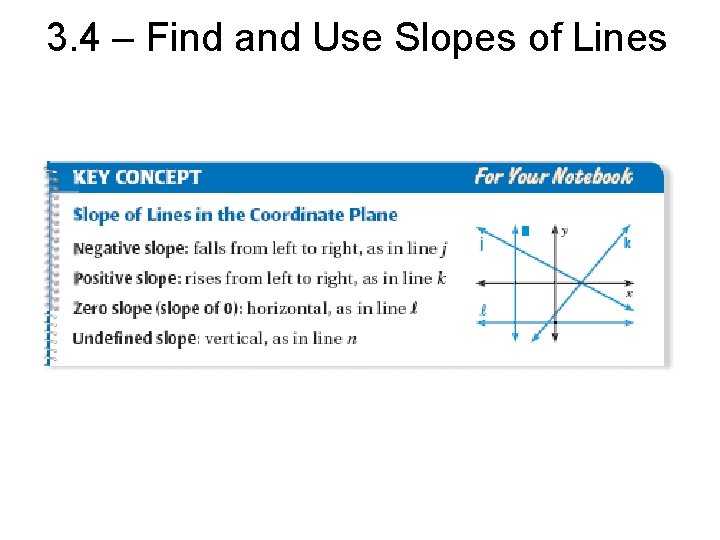3. 4 – Find and Use Slopes of Lines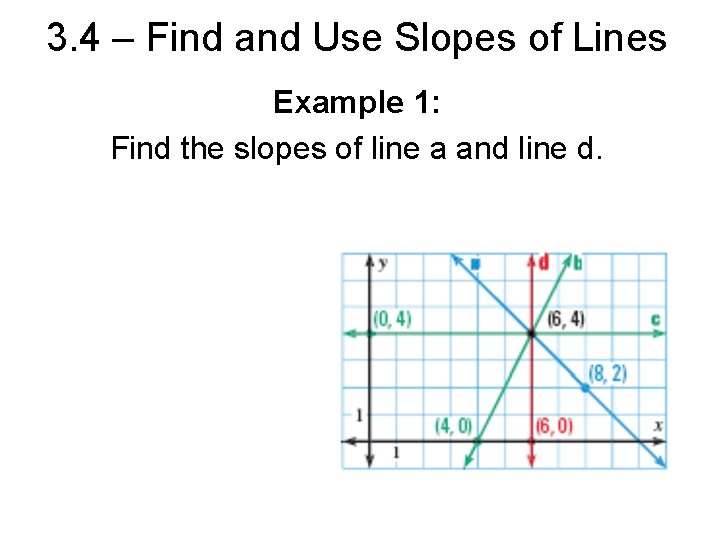3. 4 – Find and Use Slopes of Lines Example 1: Find the slopes of line a and line d.3. 4 – Find and Use Slopes of Lines Example 2: Find the slopes of line b and line c.3. 4 – Find and Use Slopes of Lines Comparing Slopes When two lines intersect in a coordinate plane, the steeper line has the slope with greater absolute value. You can also compare slopes to tell whether two lines are parallel or perpendicular.3. 4 – Find and Use Slopes of Lines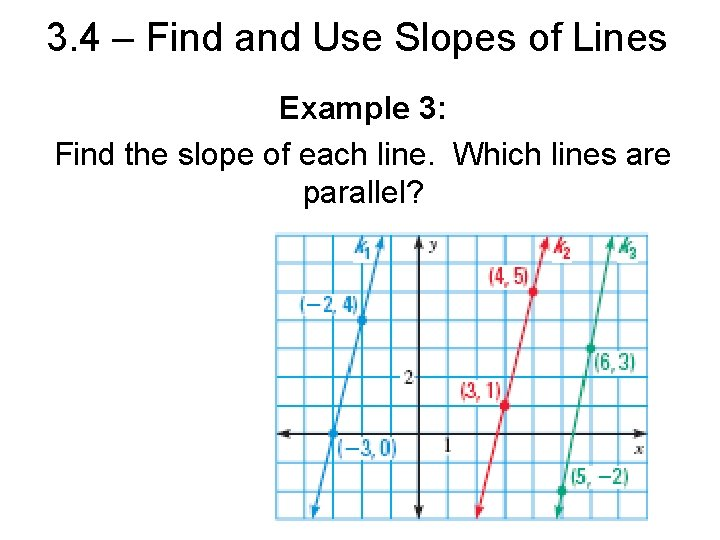3. 4 – Find and Use Slopes of Lines Example 3: Find the slope of each line. Which lines are parallel?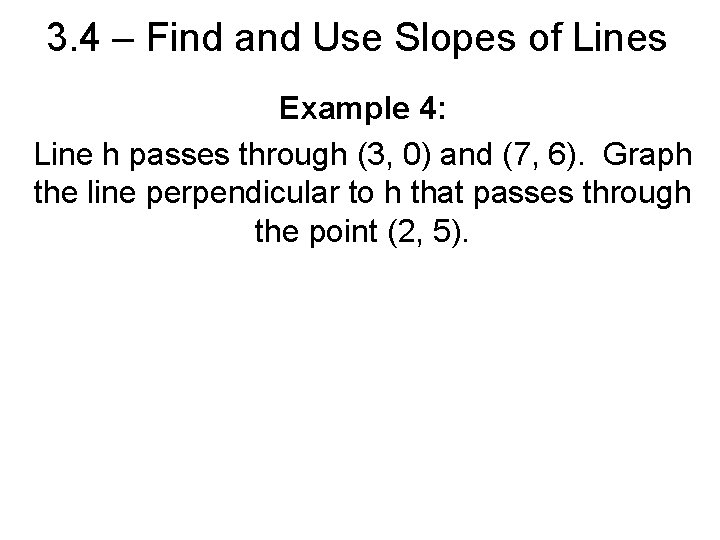3. 4 – Find and Use Slopes of Lines Example 4: Line h passes through (3, 0) and (7, 6). Graph the line perpendicular to h that passes through the point (2, 5).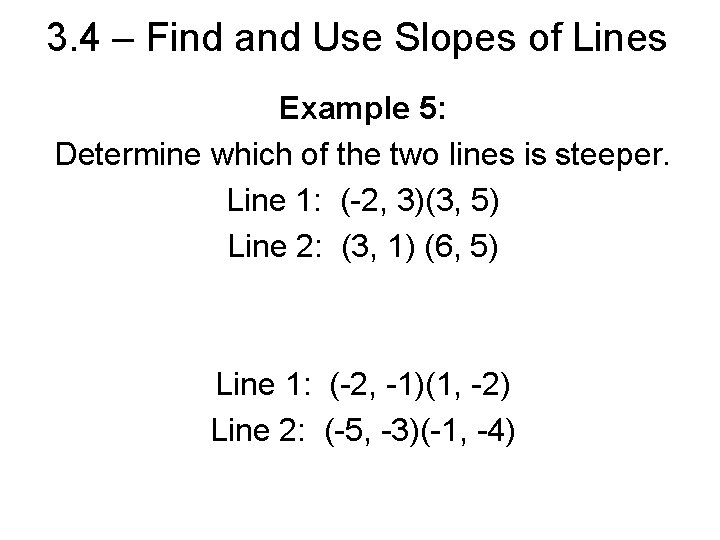3. 4 – Find and Use Slopes of Lines Example 5: Determine which of the two lines is steeper. Line 1: (-2, 3)(3, 5) Line 2: (3, 1) (6, 5) Line 1: (-2, -1)(1, -2) Line 2: (-5, -3)(-1, -4)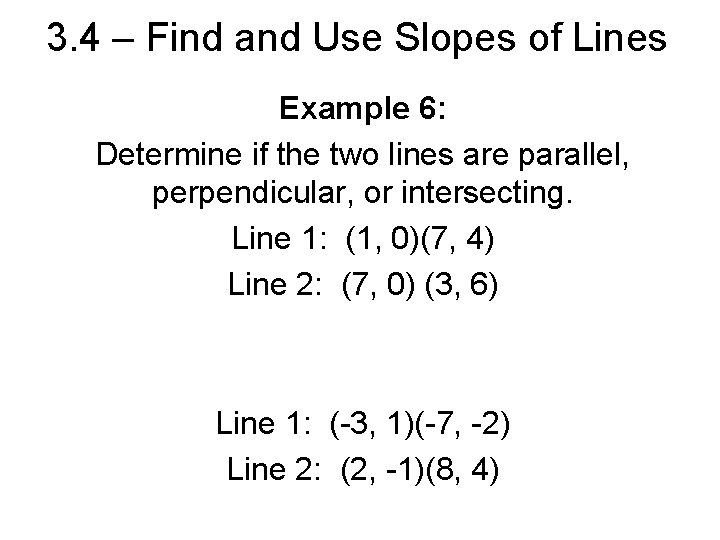3. 4 – Find and Use Slopes of Lines Example 6: Determine if the two lines are parallel, perpendicular, or intersecting. Line 1: (1, 0)(7, 4) Line 2: (7, 0) (3, 6) Line 1: (-3, 1)(-7, -2) Line 2: (2, -1)(8, 4)3. 4 – Find and Use Slopes of Lines Example 7: